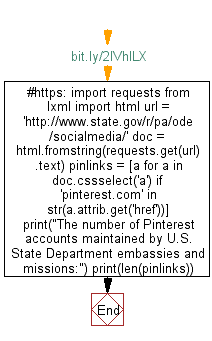# Python Web Scraping: Get the number of Pinterest accounts maintained by US state department embassies and missions

## Python Web Scraping: Exercise-15 with Solution

Write a Python program to get the number of Pinterest accounts maintained by U.S. State Department embassies and missions.

Source: https://www.state.gov/r/pa/ode/socialmedia/

Sample Solution:-

Python Code:

``````#https://bit.ly/2lVhlLX
import requests
from lxml import html
url = 'http://www.state.gov/r/pa/ode/socialmedia/'
doc = html.fromstring(requests.get(url).text)
pinlinks = [a for a in doc.cssselect('a') if 'pinterest.com' in str(a.attrib.get('href'))]
print("The number of Pinterest accounts maintained by U.S. State Department embassies and missions:")
```
```

Output

```The number of Pinterest accounts maintained by U.S. State Department embassies and missions:
3
```

Flowchart:Python Code Editor:

Have another way to solve this solution? Contribute your code (and comments) through Disqus.

What is the difficulty level of this exercise?

﻿

## Python: Tips of the Day

Merges two or more lists into a list of lists, combining elements from each of the input lists based on their positions

Example:

```def tips_merge(*args, fill_value=None):
max_length = max([len(lst) for lst in args])
result = []
for i in range(max_length):
result.append([
args[k][i] if i < len(args[k])
else fill_value for k in range(len(args))
])
return result
print(tips_merge(['x', 'y'], [1, 2], [True, False]))
print(tips_merge(['x'], [1, 2], [True, False]))
print(tips_merge(['x'], [1, 2], [True, False], fill_value = '_'))
```

Output:

```[['x', 1, True], ['y', 2, False]]
[['x', 1, True], [None, 2, False]]
[['x', 1, True], ['_', 2, False]]
```